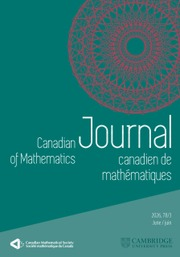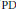Home
Hostname: page-component-6f6fcd54b-dgkch Total loading time: 0.376 Render date: 2021-05-12T02:56:25.385Z Has data issue: true Feature Flags: {}Canadian Journal of Mathematics

# Partial Differential Hamiltonian Systems

Published online by Cambridge University Press:  20 November 2018

Corresponding

## Abstract

We define partial differential ($\text{PD}$ in the following), i.e., field theoretic analogues of Hamiltonian systems on abstract symplectic manifolds and study their main properties, namely,$\text{PD}$ Hamilton equations,$\text{PD}$ Noether theorem,$\text{PD}$ Poisson bracket, etc. Unlike the standard multisymplectic approach to Hamiltonian field theory, in our formalism, the geometric structure (kinematics) and the dynamical information on the “phase space” appear as just different components of one single geometric object.

## Keywords

Type
Research Article
Information
Canadian Journal of Mathematics , 01 October 2013 , pp. 1164 - 1200

## References

 Aldaya, V., and de Azcárraga, J., Higher Order Hamiltonian Formalism in Field Theory. J. Phys. A: Math. Gen. 13(1982), 2545. http://dx.doi.org/10.1088/0305-4470/13/8/004 CrossRefGoogle Scholar
 Alonso-Blanco, R. J. and Vinogradov, A. M., Green Formula and Legendre Transformation. Acta Appl. Math. 83(2004), 149. http://dx.doi.org/10.1023/B:ACAP.0000035594.33327.71 CrossRefGoogle Scholar
 Awane, A., k-Symplectic Structures. J. Math. Phys. 32(1992), 4046. http://dx.doi.org/10.1063/1.529855 CrossRefGoogle Scholar
 Bocharov, A. V., Chetverikov, V. N., Duzhin, S. V., Khor’kova, N. G., Krasil’shchik, I. S., Samokhin, A. V., Torkhov, Yu. N., Verbovetsky, A. M., and Vinogradov, A. M., Symmetries and Conservation Laws for Differential Equations of Mathematical Physics. Transl. Math. Mon. 182, Amer. Math. Soc., Providence, 1999.Google Scholar
 Bridges, T. J., Multi-symplectic Structures and Wave Propagation. Math. Proc. Camb. Philos. Soc. 121(1997), 147. http://dx.doi.org/10.1017/S0305004196001429 CrossRefGoogle Scholar
 Bridges, T. J. and Reich, S., Multi-symplectic Integrators: Numerical Schemes for Hamiltonian PDEs that Preserve Symplecticity. Phys. Lett. A284(2001), 184. http://dx.doi.org/10.1016/S0375-9601(01)00294-8 CrossRefGoogle Scholar
 Cantrijn, F. and Ibort, A.. de Lóen, M., On the Geometry of Multisymplectic Manifolds. J. Austral. Math. Soc. Ser. A 66(1999), 303. http://dx.doi.org/10.1017/S1446788700036636 CrossRefGoogle Scholar
 Cotter, C. J., Holm, D. D., and Hydon, P. E., Multisymplectic Formulation of Fluid Dynamics Using the Inverse Map. Proc. Roy. Soc. A463(2007), 2671. chttp://dx.doi.org/10.1098/rspa.2007.1892 CrossRefGoogle Scholar
 Crnković, C. and Witten, E., Covariant Description of Canonical Formalism in Geometrical Theories. In: Three Hundred Years of Gravitation (eds. S.W. Hawking andW. Israel), Cambridge University Press, Cambridge, 1987, 676.Google Scholar
 Dedecker, P., On the Generalization of Symplectic Geometry to Multiple Integrals in the Calculus of Variations. Lecture Notes in Math. 570, Springer, Berlin, 1977, 395.Google Scholar
 de Lecn, M., Marín-Solano, J., and Marrero, J. C., The Constraint Algorithm in the Jet Formalism. Diff. Geom. Appl. 6(1996), 275. http://dx.doi.org/10.1016/0926-2245(96)82423-5 CrossRefGoogle Scholar
 de León, M., A Geometrical Approach to Classical Field Theories: a Constraint Algorithm for Singular Theories. Math. Appl. 350, Kluwer, Dordrecht, 1996, 291.Google Scholar
 de León, M., Marín-Solano, J., Marrero, J. C., Mu˜ñoz-Lecanda, M. C., and Román-Roy, N., Singular Lagrangian on Jet Bundles. Fort. Phys. 50(2002), 103. arxiv:math-ph/0105012Google Scholar
 de León, M., Martin de Diego, D., and Santamaria-Merino, A., Symmetries in Classical Field Theory. Int. J. Geom. Methods Mod. Phys. 1(2004), 651. http://dx.doi.org/10.1142/S0219887804000290 CrossRefGoogle Scholar
 de León, M., Marín-Solano, J., Marrero, J. C., Mu˜ñoz-Lecanda, M. C., and Román-Roy, N., Pre-Multisymplectic Constraint Algorithm for Field Theories. Int. J. Geom. Methods Mod. Phys. 2(2005), 839. http://dx.doi.org/10.1142/S0219887805000880 CrossRefGoogle Scholar
 Dubrovin, B. A. and Novikov, S. P., Hamiltonian Formalism of One-Dimensional Systems of Hydrodynamic Type and the Bogolyubov–Whitham Averaging Method. Dokl. Akad. Nauk SSSR 270(1983), 781785; Soviet Math. Dokl. 27(1983), 665.Google Scholar
 Dubrovin, B. A., On Poisson Brackets of Hydrodynamic Type. Dokl. Akad. Nauk SSSR 279(1984), 294–297; Soviet Math. Dokl. 30(1984), 651.Google Scholar
 Echeverría-Enríquez, A., Mu˜ñoz-Lecanda, M. C., and N. Román-Roy, , Geometry of Multisymplectic Hamiltonian First-Order Field Theories. J. Math. Phys. 41(2000), 7402. http://dx.doi.org/10.1063/1.1308075 CrossRefGoogle Scholar
 Echeverría-Enríquez, A., Geometry of Lagrangian First-Order Classical Field Theories. Forts. Phys. 44(1996), 235. http://dx.doi.org/10.1002/prop.2190440304 CrossRefGoogle Scholar
 Forger, M. and Gomes, L., Multisymplectic and Polysymplectic Structures on Fiber Bundles. arxiv:0708.1596Google Scholar
 Forger, M., Paufler, C., and Römer, H., The Poisson Bracket for Poisson Forms in Multisymplectic Field Theory. Rev. Math. Phys. 15(2003), 705. http://dx.doi.org/10.1142/S0129055X03001734 CrossRefGoogle Scholar
 Forger, M., A General Construction of Poisson Brackets on Exact Multisymplectic Manifolds. Rep. Math. Phys. 51(2003), 187. http://dx.doi.org/10.1016/S0034-4877(03)80012-5 CrossRefGoogle Scholar
 Forger, M., Hamiltonian Multivector Fields and Poisson Forms in Multisymplectic Field Theory. J. Math. Phys. 46(2005), 112903. http://dx.doi.org/10.1063/1.2116320 CrossRefGoogle Scholar
 Forger, M. and Römer, H., A Poisson Bracket on Multisymplectic Phase Space. Rep. Math. Phys. 48(2001), 211. http://dx.doi.org/10.1016/S0034-4877(01)80081-1 CrossRefGoogle Scholar
 Forger, M. and Romero, S., Covariant Poisson Brackets in Geometric Field Theory. Commun. Math. Phys. 256(2005), 375. http://dx.doi.org/10.1007/s00220-005-1287-8 CrossRefGoogle Scholar
 Goldshmidt, H. and Sternberg, S., The Hamilton–Cartan Formalism in the Calculus of Variations. Ann. Inst. Fourier 23(1973), 203. http://dx.doi.org/10.5802/aif.451 CrossRefGoogle Scholar
 Gotay, M. J., A Multisymplectic Approach to the KdV Equation. In: Differential Geometric Methods in Mathematical Physics (eds. K. Bleuler and M.Werner), Kluwer, Amsterdam, 1988, 295.Google Scholar
 Gotay, M. J., Isenberg, J., and Marsden, J. E., Momentum Maps and Classical Relativistic Fields. I: Covariant Field Theory. arxiv:physics/9801019Google Scholar
 Gotay, M. J., Nester, J. M., and Hinds, G., Presymplectic Manifolds and the Dirac–Bergmann Theory of Constraints. J. Math. Phys. 19(1978), 2388. http://dx.doi.org/10.1063/1.523597 CrossRefGoogle Scholar
 Grabowska, K., A Tulczyjew Triple for Classical Fields. J. Phys. A: Math. Theor. 45(2012), 145207. http://dx.doi.org/10.1088/1751-8113/45/14/145207 CrossRefGoogle Scholar
 Grabowska, K., Grabowski, J., and Urbański, P., AV-Differential Geometry: Poisson and Jacobi Structures. J. Geom. Phys. 52(2004), 398. http://dx.doi.org/10.1016/j.geomphys.2004.04.004 CrossRefGoogle Scholar
 Grabowska, K., AV-Differential Geometry: Euler–Lagrange Equations. J. Geom. Phys. 57(2007), 1984. http://dx.doi.org/10.1016/j.geomphys.2007.04.003 CrossRefGoogle Scholar
 Gracia, X., Martin, R., and Román-Roy, N., Constraint Algorithm for k-Presymplectic Hamiltonian Systems. Application to Singular Field Theories. Int. J. Geom. Methods Mod. Phys. 6(2009), 851. http://dx.doi.org/10.1142/S0219887809003795 CrossRefGoogle Scholar
 Hélein, F. and Kouneiher, J., Covariant Hamiltonian Formalism for the Calculus of Variations with Several Variables: Lepage–Dedecker versus De Donder–Weyl. Adv. Theor. Math. Phys. 8(2004), 565.CrossRefGoogle Scholar
 Henneaux, M. and Teitelboim, C., Quantization of Gauge Systems. Princeton University Press, Princeton, 1992.Google Scholar
 Kanatchikov, I. V., On Field Theoretic Generalization of a Poisson Algebra. Rep. Math. Phys. 40(1997), 225. http://dx.doi.org/10.1016/S0034-4877(97)85919-8 CrossRefGoogle Scholar
 Kijowski, J., A Finite-Dimensional Canonical Formalism in the Classical Field Theory. Commun. Math. Phys. 30(1973), 99. http://dx.doi.org/10.1007/BF01645975 CrossRefGoogle Scholar
 Kijowski, J. and Szczyrba, W., Multisymplectic Manifolds and the Geometrical Construction of the Poisson Bracket in Field Theory. In: Géométrie Symplectique et Physique Mathématique (ed. J.-M. Souriau), Colloq. Internat. C. N. R. S. 237(1975), 347.Google Scholar
 Kolář, I., A Geometric Version of the Higher Order Hamilton Formalism in Fibered Manifolds. J. Geom. Phys. 1(1984), 127. http://dx.doi.org/10.1016/0393-0440(84)90007-X CrossRefGoogle Scholar
 Krupkova, O., Hamiltonian Field Theory. J. Geom. Phys. 43(2002), 93. http://dx.doi.org/10.1016/S0393-0440(01)00087-0 CrossRefGoogle Scholar
 Lee, J. and Wald, R., Local Symmetries and Constraints. J. Math. Phys. 31(1990), 725. http://dx.doi.org/10.1063/1.528801CrossRefGoogle Scholar
 Marsden, J., Pekarsky, S., Shkoller, S., and West, M., Variational Methods, Multisymplectic Geometry and Continuum Mechanics. J. Geom. Phys. 38(2001), 253. http://dx.doi.org/10.1016/S0393-0440(00)00066-8 CrossRefGoogle Scholar
 Martin, G., A Darboux Theorem for Multisymplectic Manifolds. Lett. Math. Phys. 16(1988), 133.http://dx.doi.org/10.1007/BF00402020 CrossRefGoogle Scholar
 Michor, P.W., Topics in Differential Geometry. Graduate Stud. in Math. 93, Amer. Math. Soc., Providence, 2008.Google Scholar
 Mokhov, O. I., Symplectic and Poisson Geometry on Loop Spaces of Manifolds and Nonlinear Equations. Uspekhi Mat. Nauk 53(1998), 85–192; (English) Russian Math. Surveys 53(1998), 515.http://dx.doi.org/10.4213/rm19 Google Scholar
 Moreno, G., Vinogradov, A. M., and Vitagliano, G., Integrals and Cohomology. In preparation.Google Scholar
 Paufler, C. and Römer, H., Geometry of Hamiltonian n-Vectors in Multisymplectic Field Theory. J. Geom. Phys. 44(2002), 52. http://dx.doi.org/10.1016/S0393-0440(02)00031-1 CrossRefGoogle Scholar
 Paufler, C., de Donder–Weyl Equations and Multisymplectic Geometry. Rep. Math. Phys. 49(2002), 325. http://dx.doi.org/10.1016/S0034-4877(02)80030-1 CrossRefGoogle Scholar
 Román-Roy, N., Multisymplectic Lagrangian and Hamiltonian Formalism of First-Order Classical Field Theories. SIGMA 5(2009), 100.arxiv:math-ph/0506022Google Scholar
 Saunders, D. J., Jet Fields, Connections and Second-Order Differential Equations. J. Phys. A: Math. Gen. 20(1987), 3261.http://dx.doi.org/10.1088/0305-4470/20/11/029 CrossRefGoogle Scholar
 Saunders, D. J., The Geometry of Jet Bundles. Cambridge University Press, Cambridge, 1989.Google Scholar
 Saunders, D. J., A Note on Legendre Transformations. Diff. Geom. Appl. 1(1991), 109. http://dx.doi.org/10.1016/0926-2245(91)90025-5CrossRefGoogle Scholar
 Saunders, D. J. and Crampin, M., On the Legendre Map in Higher-Order Field Theories. J. Phys. A: Math. Gen. 23(1990), 3169. http://dx.doi.org/10.1088/0305-4470/23/14/016 CrossRefGoogle Scholar
 Shadwick, W. F., The Hamiltonian Formulation of Regular r-th Order Lagrangian Field Theories. Lett. Math. Phys. 6(1982), 409.http://dx.doi.org/10.1007/BF00405859 CrossRefGoogle Scholar
 Vinogradov, A. M., The–Spectral Sequence, Lagrangian Formalism and Conservation Laws I, II. J. Math. Anal. Appl. 100(1984), 1.http://dx.doi.org/10.1016/0022-247X(84)90071-4 Google Scholar
 Vitagliano, L., Secondary Calculus and the Covariant Phase Space. J. Geom. Phys. 59(2009), 426.http://dx.doi.org/10.1016/j.geomphys.2008.12.001 CrossRefGoogle Scholar
 Vitagliano, L., The Lagrangian–Hamiltonian Formalism for Higher Order Field Theories. J. Geom. Phys. 60(2010), 857.http://dx.doi.org/10.1016/j.geomphys.2010.02.003 CrossRefGoogle Scholar
 Zuckerman, G. J., Action Principles and Global Geometry. In: Mathematical Aspects of String Theory (ed. S. T. Yau),World Scientific, Singapore, 1987, 259.CrossRefGoogle ScholarYou have Access

# Send article to Kindle

Note you can select to send to either the @free.kindle.com or @kindle.com variations. ‘@free.kindle.com’ emails are free but can only be sent to your device when it is connected to wi-fi. ‘@kindle.com’ emails can be delivered even when you are not connected to wi-fi, but note that service fees apply.

Find out more about the Kindle Personal Document Service.

Partial Differential Hamiltonian Systems
Available formats
×

# Send article to Dropbox

To send this article to your Dropbox account, please select one or more formats and confirm that you agree to abide by our usage policies. If this is the first time you use this feature, you will be asked to authorise Cambridge Core to connect with your <service> account. Find out more about sending content to Dropbox.

Partial Differential Hamiltonian Systems
Available formats
×

# Send article to Google Drive

To send this article to your Google Drive account, please select one or more formats and confirm that you agree to abide by our usage policies. If this is the first time you use this feature, you will be asked to authorise Cambridge Core to connect with your <service> account. Find out more about sending content to Google Drive.

Partial Differential Hamiltonian Systems
Available formats
×
×